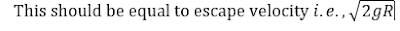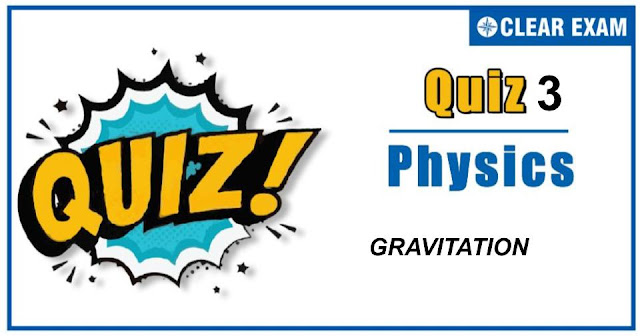## [LATEST]\$type=sticky\$show=home\$rm=0\$va=0\$count=4\$va=0

As per analysis for previous years, it has been observed that students preparing for NEET find Physics out of all the sections to be complex to handle and the majority of them are not able to comprehend the reason behind it. This problem arises especially because these aspirants appearing for the examination are more inclined to have a keen interest in Biology due to their medical background.

Furthermore, sections such as Physics are dominantly based on theories, laws, numerical in comparison to a section of Biology which is more of fact-based, life sciences, and includes substantial explanations. By using the table given below, you easily and directly access to the topics and respective links of MCQs. Moreover, to make learning smooth and efficient, all the questions come with their supportive solutions to make utilization of time even more productive. Students will be covered for all their studies as the topics are available from basics to even the most advanced.

Q1. Kelper discovered
•  Laws of motion
•  Laws of rotational motion
•  Laws of planetary motion
•  Laws of curvilinear motion
Solution

Q2.If r represents the radius of the orbit of a satellite of mass m moving around a planet of mass M, the velocity of the satellite is given by
•  v2=gMr
•  v2=GMmr
•  v=GMr
•  v2=GMr
Solution

Q3.  The ratio ggh,where g and gh are the accelerations due to gravity at the surface of the earth and at a height h above the earth's surface respectively,is
•   1+hR2
•  1+Rh2
•  Rh2
•  hR2
Solution

Q4. To an astronaut in a spaceship,the sky appears
•  Black
•  White
•  Green
•  Blue
Solution

Q5.In planetary motion the areal velocity of position vector of a planet depends on angular velocity (ω) and the distance of the planet from sun (r). If so the correct relation for areal velocity is
Solution
Q6. Earth binds the atmosphere because of
•  Gravity
•  Oxygen between earth and atmosphere
•  Both(a) and (b)
•  None of the above
Solution

Q7.A point mass is placed inside a thin spherical shell of radius R and mass M at a distance R/2 from the centre of the shell. The gravitational force exerted by the shell on the point mass is
•  GM2R2
•  -GM2R2
•  Zero
•  GRM4R2
Solution

Gravitational field inside hollow sphere will be zero.

Q8.A man weighs 80 kg on earth surface. The height above ground where he will weigh 40kg, is (radius of earth is 6400 km)
•  0.31 times r
•  0.41 times r
•  0.51 times r
•  0.61 times r
Solution

Q9.A particle falls towards earth from infinity. It’s velocity on reaching the earth would be
•  Infinity
•  2gR
•  2gR
•  Zero
SolutionQ10. What is the height the weight of body will be the same as at the same depth from the surface of the earth? Radius of earth is R
•  R2
•  5R-R
•  5R-R2
•  3R-R2
Solution## Want to know more

Want to Know More
Please fill in the details below:

## Latest NEET Articles\$type=three\$c=3\$author=hide\$comment=hide\$rm=hide\$date=hide\$snippet=hide

Name

ltr
item
Best IIT JEE Coaching Institute in Delhi | NEET Coaching Institute in Delhi: GRAVITATION QUIZ-3
GRAVITATION QUIZ-3